# NEET Chapter - The Solid State

Get plus subscription and access unlimited live and recorded courses## Introduction to The Solid State

In this chapter, we'll look at alternative particle configurations that can result in a variety of structures. The relationship between the nature of interactions within constituent particles and a variety of solid properties will also be investigated. It would also be explained how these qualities are affected by structural defects or by the presence of impurities in trace amounts.

This chapter is one of the easiest, most significant and scoring chapters for competitive tests like NEET, and mastering it will help you score well in the exams.

### Important Topics of The Solid State:

• Amorphous Solid

• Difference Between Crystalline and Amorphous Solid

• Classification of Crystalline Solids

• Crystal Lattices and Unit Cell

• Unit Cell Packing Efficiency

• Imperfections or Defects in a Solid

### Explanation

Pseudo solids

Pseudo solids are the types of solids which appear of being solid but still they act like liquid as they have a tendency to flow. Glass is an example of pseudo solids.

Anisotropy

Anisotropy is the quality of exhibiting different properties when measured along axes in different directions.

Isotropy

Isotropy is the quality of exhibiting the same properties when measured along axes in different directions.

Bravais lattice

The 14 three dimensional possible configuration in which atoms, ions or molecules are arranged in a crystal.

### Solid-State

• Properties of Solids:

The following are the characteristics of solids:

• They have fixed shape, mass and volume.

• The intermolecular force of attraction is strong.

• The intermolecular distance between the constituent particles of solids is short.

• They are rigid and incompressible.

• They have high density.

• Classification of Solids:

Solids are classified on the basis of the following properties

• On the basis of the arrangement of constituent particles and different properties:

• Amorphous Solids

• Crystalline Solids

• On the basis of bonding:

• Molecular Solids- They are further classified into polar, non-polar and  hydrogen-bonded.

• Ionic Solids

• Metallic Solids

• Covalent or network Solids

### Amorphous and Crystalline Solids

Amorphous solids have constituent particles arranged in the crystal in an irregular pattern whereas in crystalline solids the constituents particles are arranged in a regular pattern. This leads to the difference in the properties of amorphous and crystalline solids.

 Properties Crystalline Solids Amorphous Solid Range of arrangement of constituent particles Long-range order Short-range order Shape Regular or definite shape Irregular shape Melting Point Definite or sharp melting point. Melts over a range of temperatures. Cleavage property Split into two pieces with regular surfaces Split into two pieces with irregular surfaces Anisotropy They are anisotropic. They are isotropic. Nature True solids Pseudo solids or super cooled solid.

### Types of Crystalline  Solids on the Basis of Bonding

 Type of Solid Constituent Particles Bonding or Attractive Forces Examples Physical  Nature Electrical Conductivity Melting Point Molecular Solids:-Polar-Non-polar-Hydrogen   bonded Molecules -DIspersion or london forces-Dipole-diploe interaction- Hydrogen Bonding - Ar, H2, CCl4, I2- HCl, SO2- H2O(ice) -Soft-Soft-Hard -Insulator-Insulator-Insulator -Very low-low-low Ionic Solids Ions Electrostatic/ Coulombic NaCl, HBr, MgCl2 Hard but brittle Insulators in the solid state but conductors in molten of aqueous state High Metallic Solids Positive ion in delocalised in the sea of free electrons Metallic Bonding Fe, Cu, Au, Ag Hard but malleable and ductile ConductorIn solid and molten state Very High Covalent or network Solids Atoms Covalent Bonding C(diamond), C (graphite),SiO2(quartz), AIN Hard except graphite Insulator except graphite, which is a conductor Very High

### Graphite and Diamond:

Graphite and diamond are the covalent solids, but they are different in most of the properties as shown below:

 Properties Diamond Graphite Physical property Hardest covalent solid Soft covalent solid Conductivity Insulator Conductor C-C bond length C-C bond length is 154 pm. C-C bond length is 142 pm but the distance is 340 pm. Structure Three-dimensional polymeric network structure.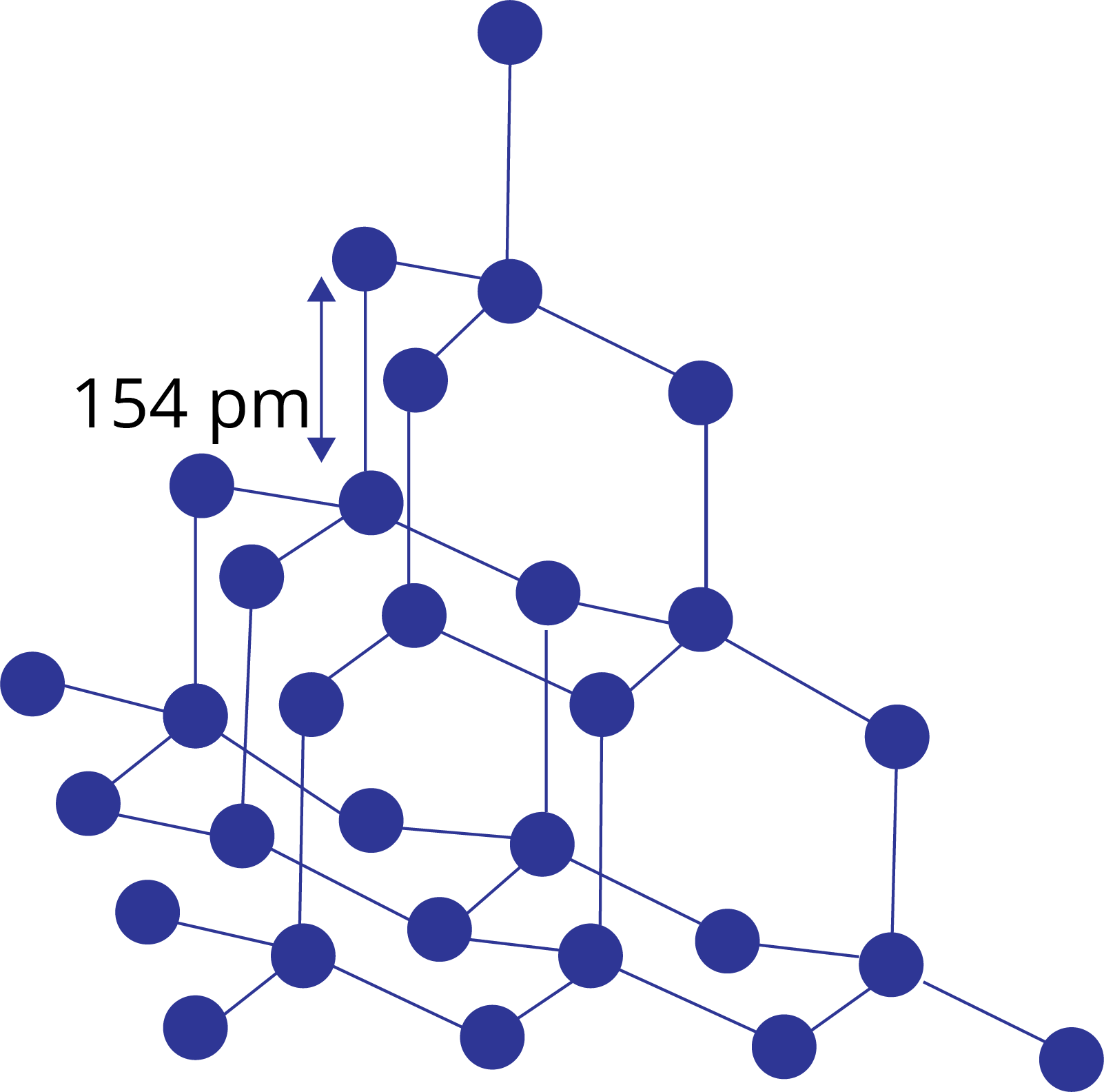Two-dimensional layered structure.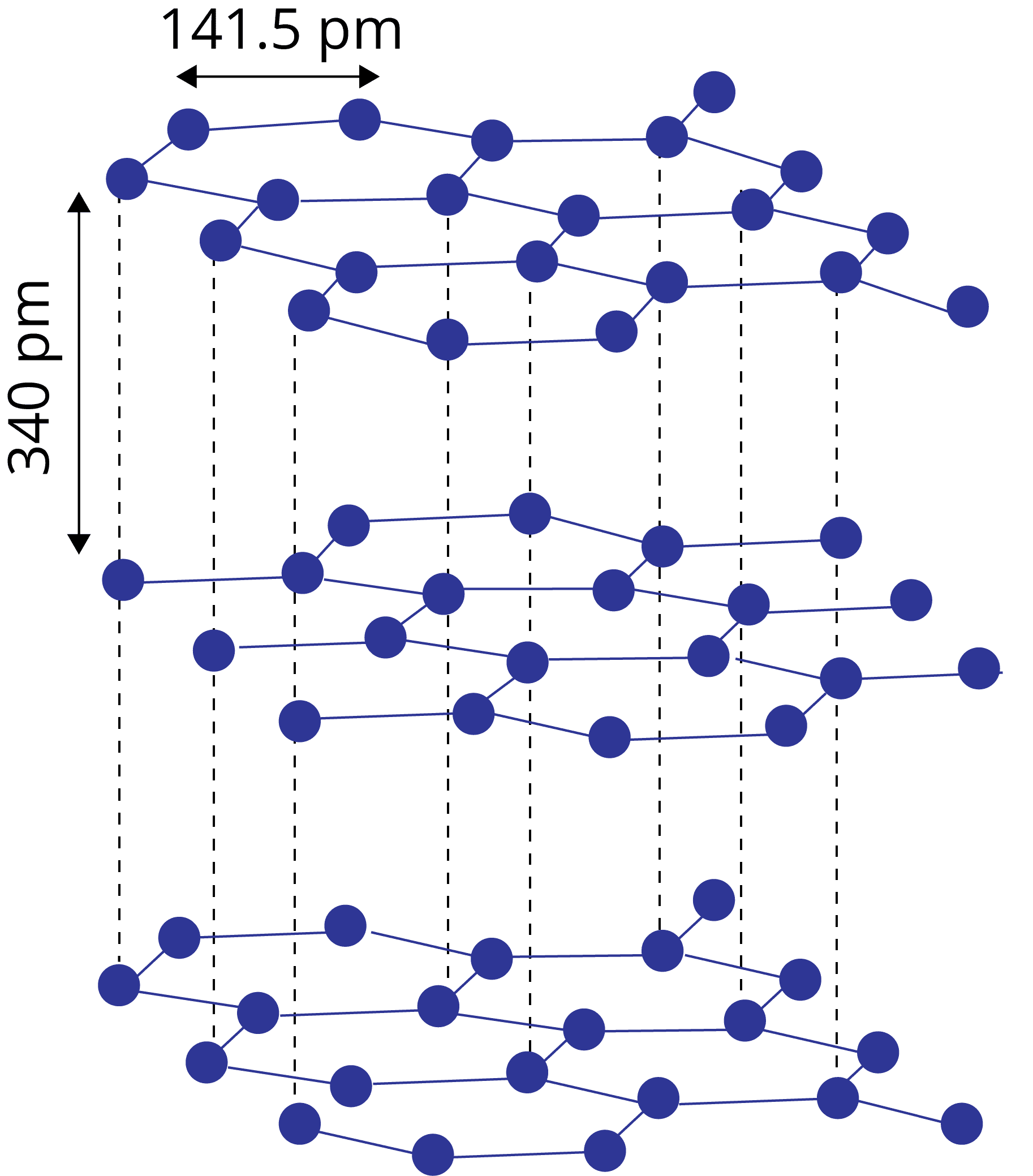### Structure of Crystalline Solids

Crystal Lattice and Unit Cell:  The regular three-dimensional spatial arrangement of atoms, ions or molecules in the solids is known as the crystal lattice.

The smallest component of a crystal lattice that is repeated in all directions to form the entire crystal lattice is referred to as a unit cell.

In a unit cell, atoms of ions or molecules are represented by the sphere and the different types of the lattice is formed due to variation in the following parameters:

• Edge lengths: a,b,c

• Interfacial angles: α, β, γ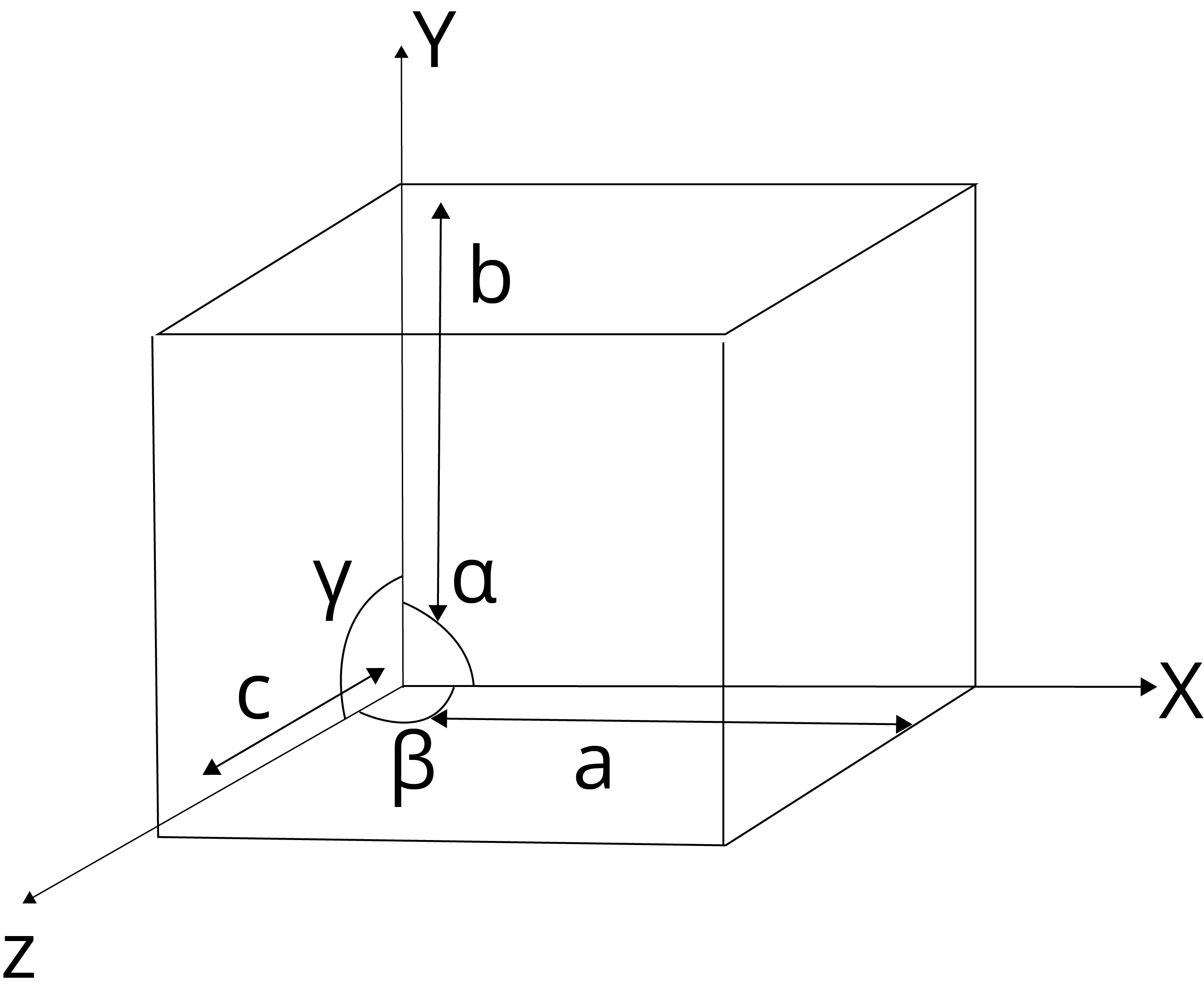Fig.: parameters of unit cell

• Primitive Unit Cell:

There are generally 7 types of unit cells based on the variation in parameters of the unit cell in which the constituent atoms of ions or molecules present at the corner of the cell are known as primitive unit cells.

 Crystal System Axial distance Axial angle Example Cubic a = b =  c α =  β =  γ = 90o NaCl, ZnS, KCl, Cu Tetragonal a = b ≠  c α =  β =  γ = 90o SiO2, white tin(Sn,), CaSO4, TiO2 Orthorhombic a ≠ b ≠  c α =  β =  γ = 90o Rhombic sulphur, KNO3, BaSO4 Monoclinic a ≠ b ≠  c α =  β  = 90o  γ ≠ 90o Monoclinic sulphur, PbCrO2, Na2SO4.10H2O Hexagonal a = b ≠  c α =  β = 90oand γ =120o Graphite, ZnO, CdS Trigonal or rhombohedral a ≠ b ≠  c α =  β =  γ ≠ 90o Calcite(CaCO3), Cinnabar(HgS) Triclinic a ≠ b ≠  c α ≠  β ≠  γ ≠ 90o K2Cr2O7, CuSO4.5H2O, H3BO3

Table: 7 Primitive Unit Cells

• Centered  Unit Cell: A centered unit cell is one that comprises one or more constituent particles in positions other than the corners in addition to those at the corners.

There are three types of centred unit cells:

• Body-Centered Unit Cells: This type of unit cell contains one constituent(atom, ion or molecule) at the body centre in addition to those present at the corners.

• Face-Centered Unit Cells: This type of unit cell contains one constituent(atom, ion or molecule) at the centre of each face in addition to those present at the corners.

• End-Centered Unit Cells: This type of unit cell contains one constituent(atom, ion or molecule) at the centre of any two opposite faces in addition to those present at the corners.

 Cubic crystal Number of atoms, their contribution at lattice positions Structure Number of atoms (Z) Relation between edge length and radius Packing efficiency Corner Body centre Face centre Simple cubic 8,⅛ X 8 = 1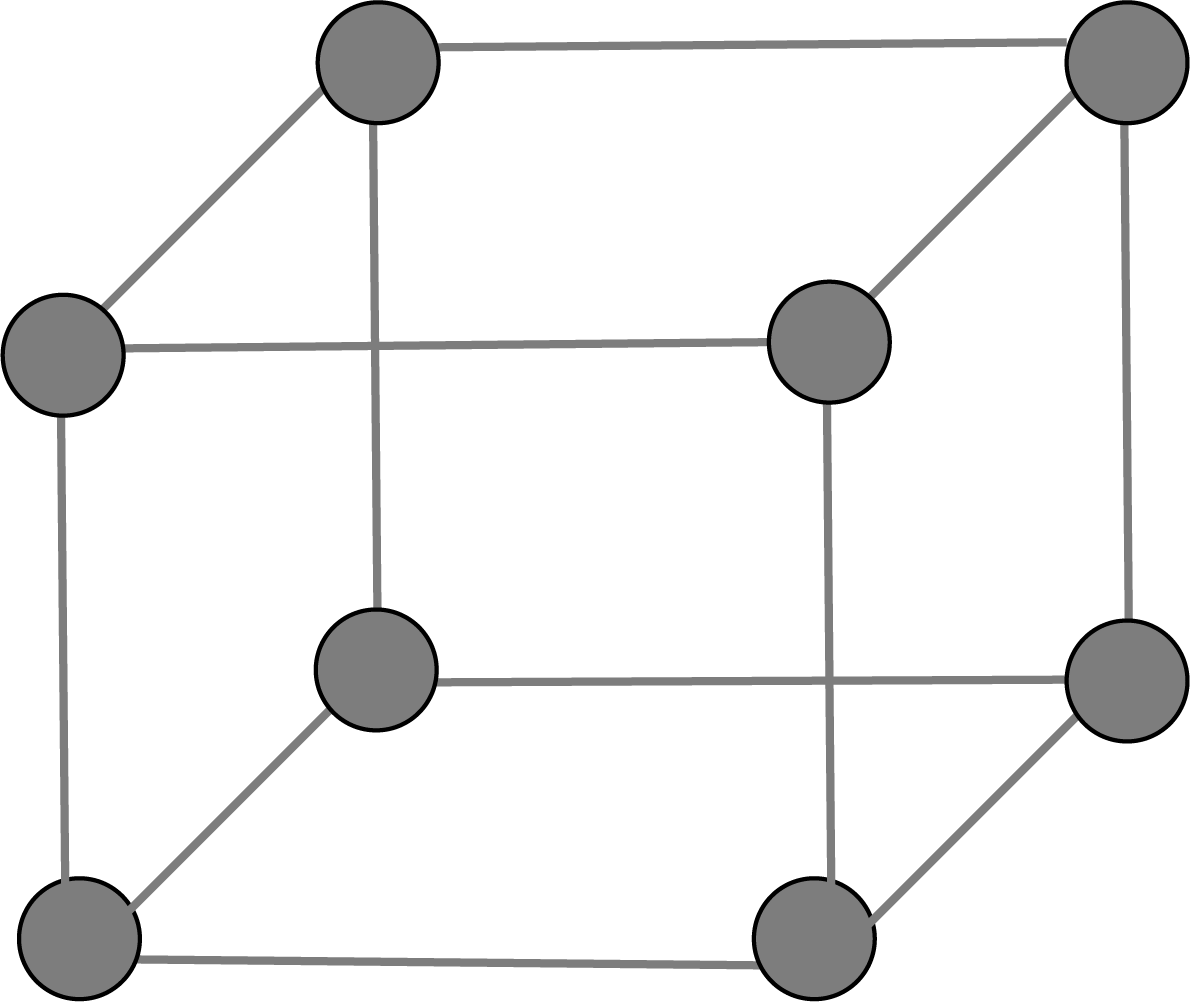1 r = a/2 52% Body-centred 8,⅛ X 8 = 1 1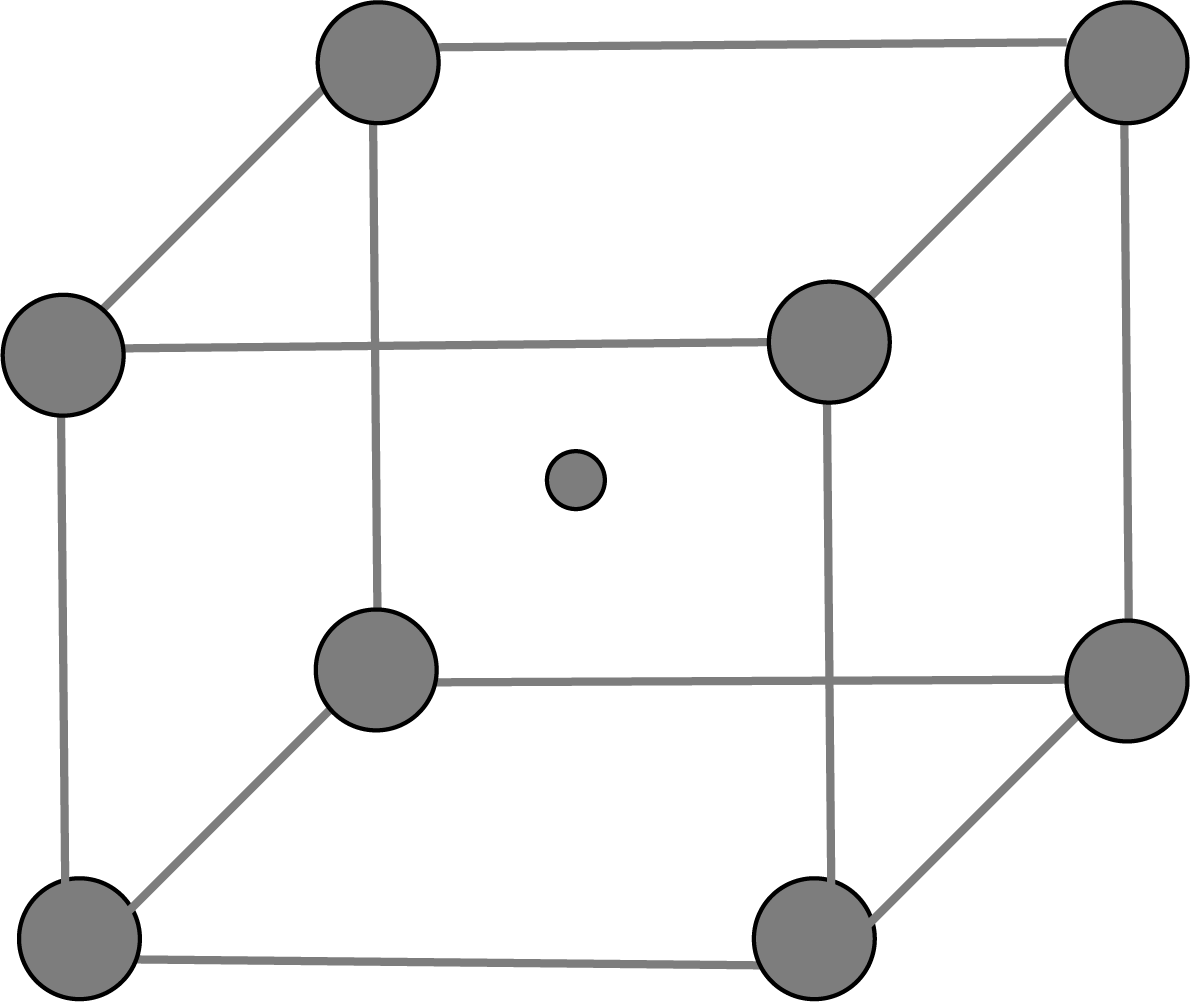2 r = $\dfrac{\sqrt{3}a}{4}$ 68% Face-centred 8,⅛ X 8 = 1 6,½  X 6 = 3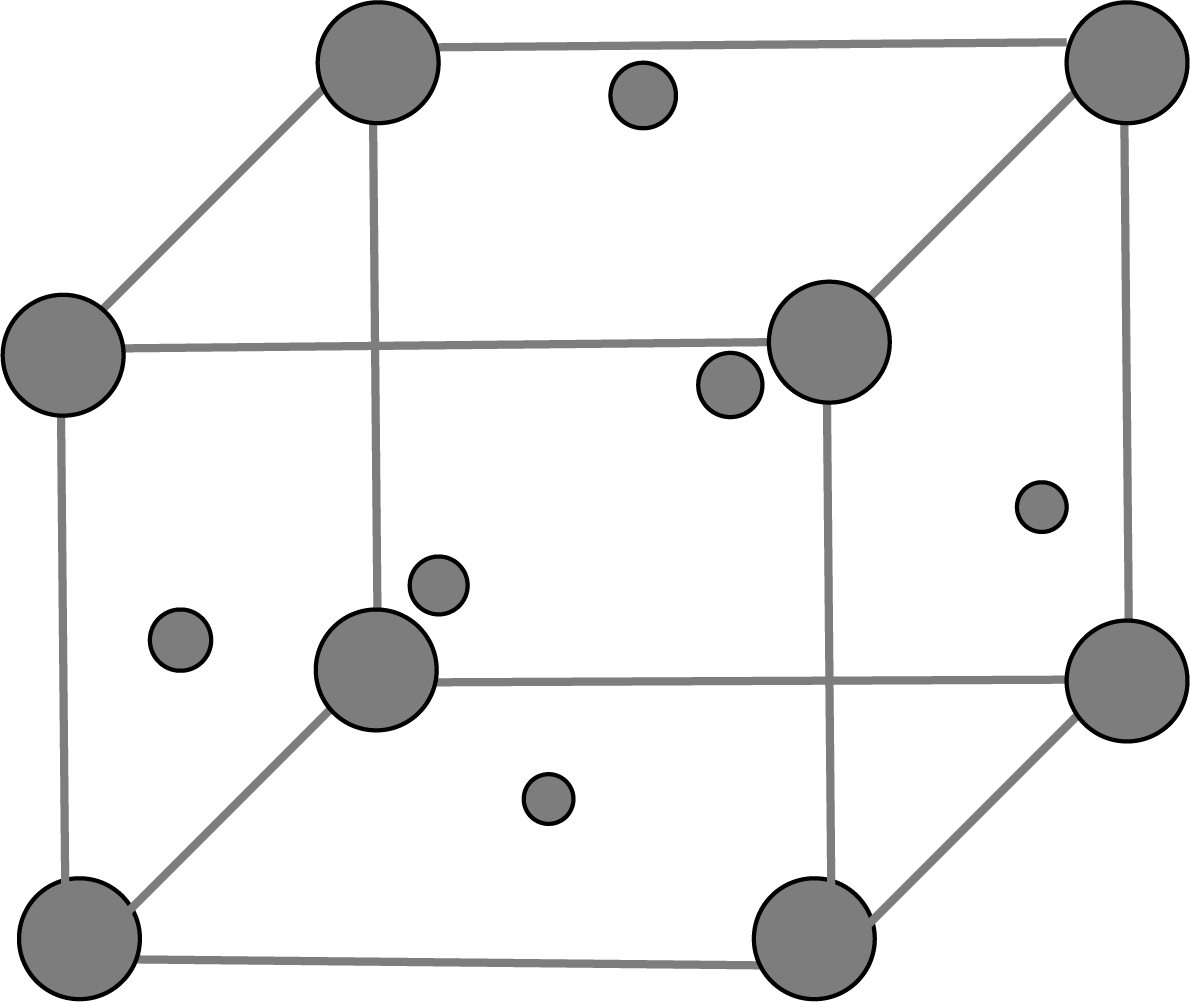4 r = $\dfrac{\sqrt{2}a}{4}$ 74%

Contribution of spherical constituents are as follows:

• Corner:  ⅛ of number of constituent particles at the corner

• Body centre: 1

• Face centre: ⅓ of number of constituent particles at the faces

### Density of Cubic Crystals

Density of cubic crystals is given by the formula:

Density,  ρ = $\dfrac{M \times Z}{a^{3}N_A}$

where M: Molar mass of solid

Z:  Total number of atom in the unit cell

A:  Edge length

### Close Packing in Solids

Suppose we have spheres of identical size that must be arranged in a single layer with the requirement that the spheres be in close contact with each other. There are two types of possible packing:

• Square packing

• Hexagonal packing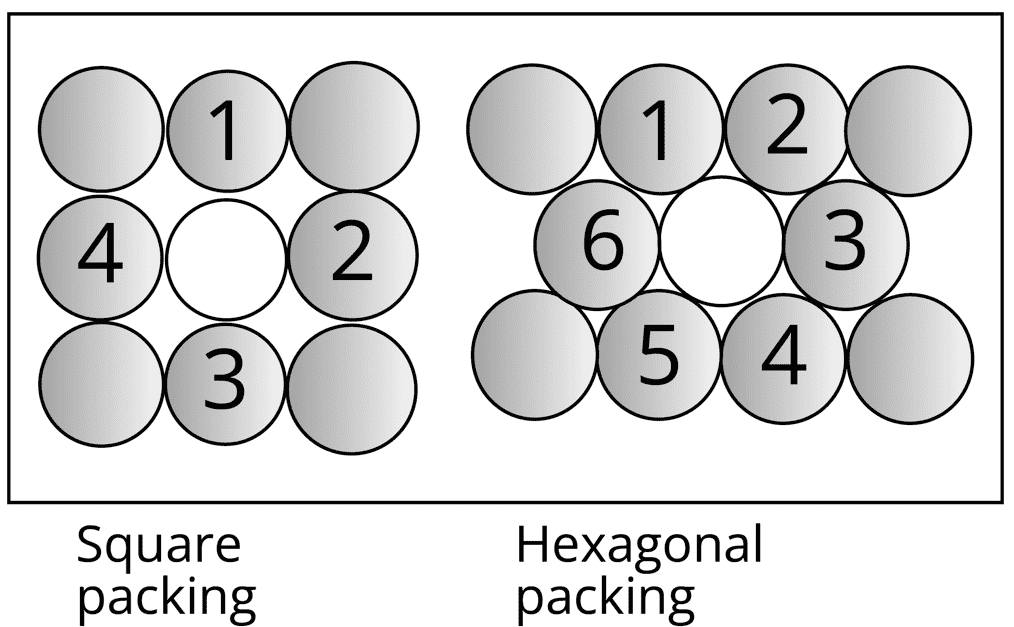Hexagonal packing is more efficient:

• In hexagonal packing, the coordination number is 6, whereas, in square packing, the coordination number is 4.

• In hexagonal packing square packing the size of the void is bigger than that of hexagonal packing.

If we add another layer to the square packing, then there are the following possibilities:

• Simple Cubic Unit Cell: A comparable layer is placed just above the foundation layer, with the second layer's spheres coming just above the first layer's spheres, and the layers are repeated. The first layer is referred to as A, and the packing is of the AA... type, and the unit cell is a simple cubic unit cell.

• BCC Unit Cell: When the spheres of the second layer are positioned in the depressions of the first layer, we get the ABAB…… packing type and the unit cell is the BCC unit cell.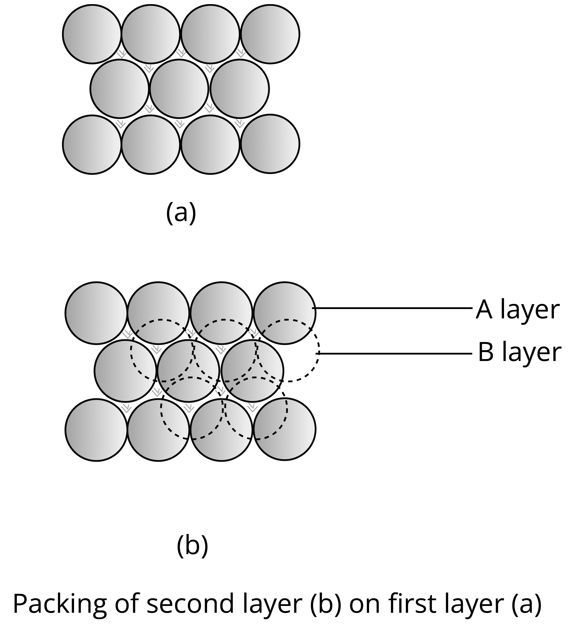The types of arrangement of spheres in hexagonal packing in the voids are:

• When the second hexagonal layer is placed in the depressions of the first hexagonal layer A, two types of voids are formed.

• X type of voids are those which are hollow and through voids of layer A and layer B while Y type of voids are those voids of layer B which are exactly above spheres of layer A.

• If the sphere of the 2nd layer is placed on Y type void, then the 1st layer is repeated and ABABAB…. type hexagonal unit cell is obtained and the packing is hexagonal close packing(HCP). The efficiency of hexagonal close packing is 74%.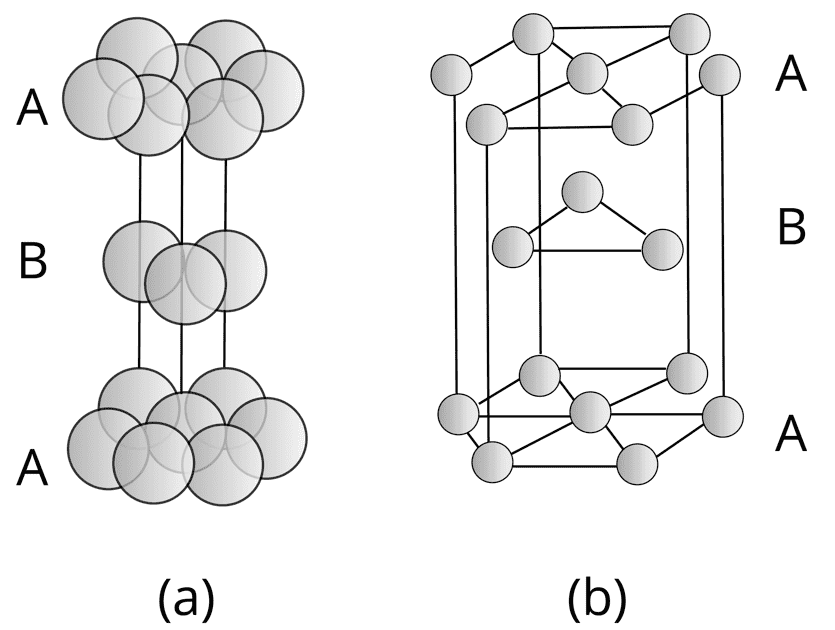• When the 3rd layer is placed on X type of void, then a new layer C is obtained, and the packing is ABCABC…. Type. The unit cell for this type of arrangement is face-centred cubic (FCC) or cubic close packing(CCP). The efficiency of FCC or CCP is 74%.

### Classification of Ionic Structures

In the ionic structure, the ion of larger size occupies the main position whereas the smaller one occupies the void position. The size is decide by the radius ratio r+/r-

 Radius ratio range x  =  r+/r- Coordination Number Shape Example x < 0.155 3 Linear BeF2 0.155 ≤ x ≤ 0.225 3 Trigonal Planar AlCl3 0.225 ≤ x ≤ 0.414 4 Tetrahedron ZnS 0.414 ≤ x ≤ 0.732 6 Octahedron NaCl 0.732 ≤ x ≤ 0.999 8 Body centered cubic CsCl

Based on the ratio range, there are five types of ionic structures:

• NaCl Type Structure:

 Figure Key Points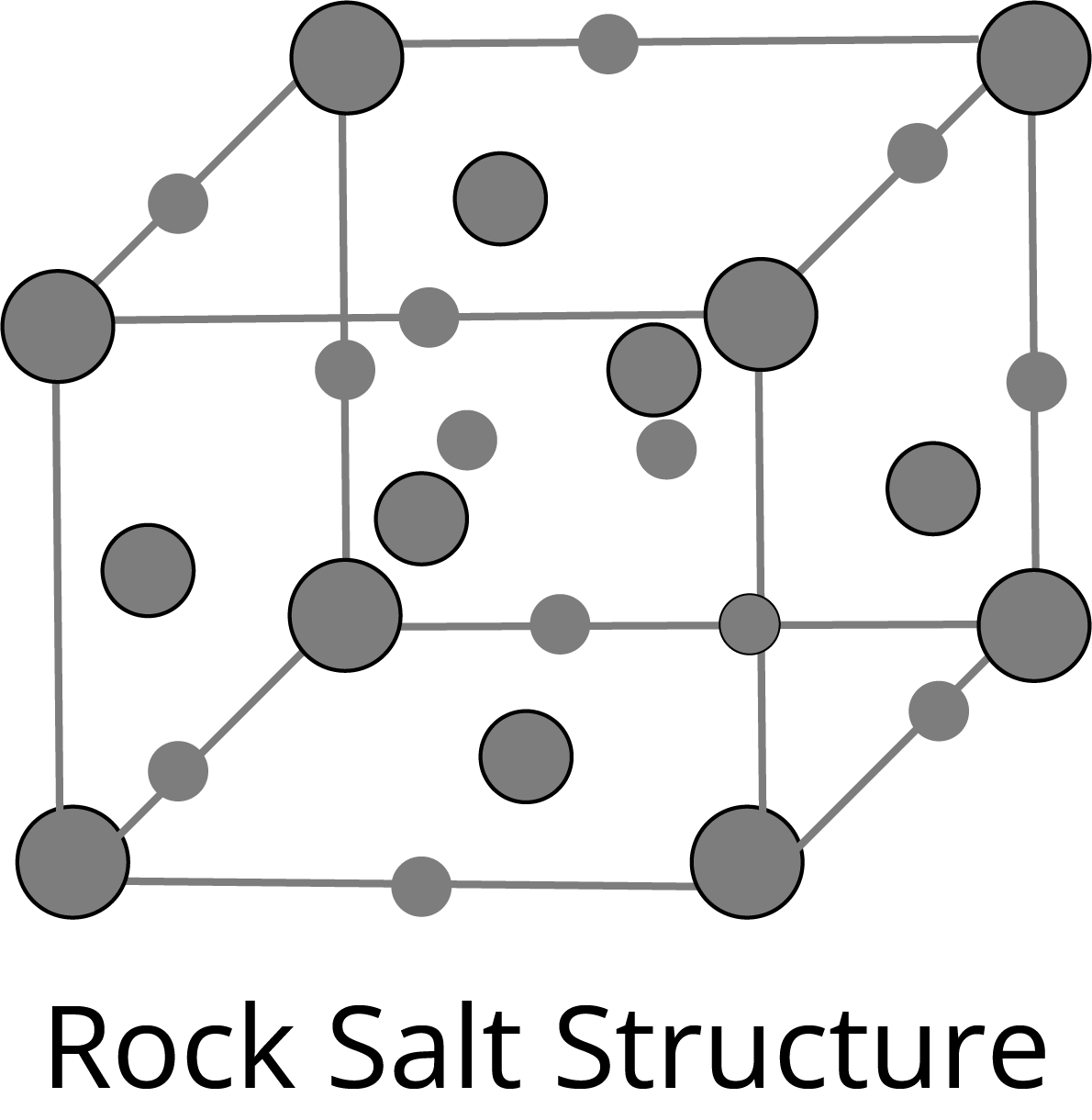Cl- occupy corners and face centres and Na+ occupy octahedral voids in FCC crystal.Effective formula=Na4Cl4Co-ordination No. of Na+=6Co-ordination No. of Cl-=6Distance b/w nearest neigbhours ($r_{Na^{+}}+r_{Cl^{-}}=\frac{a}{2}$)

• ZnS Type Structure:

 Figure Key Points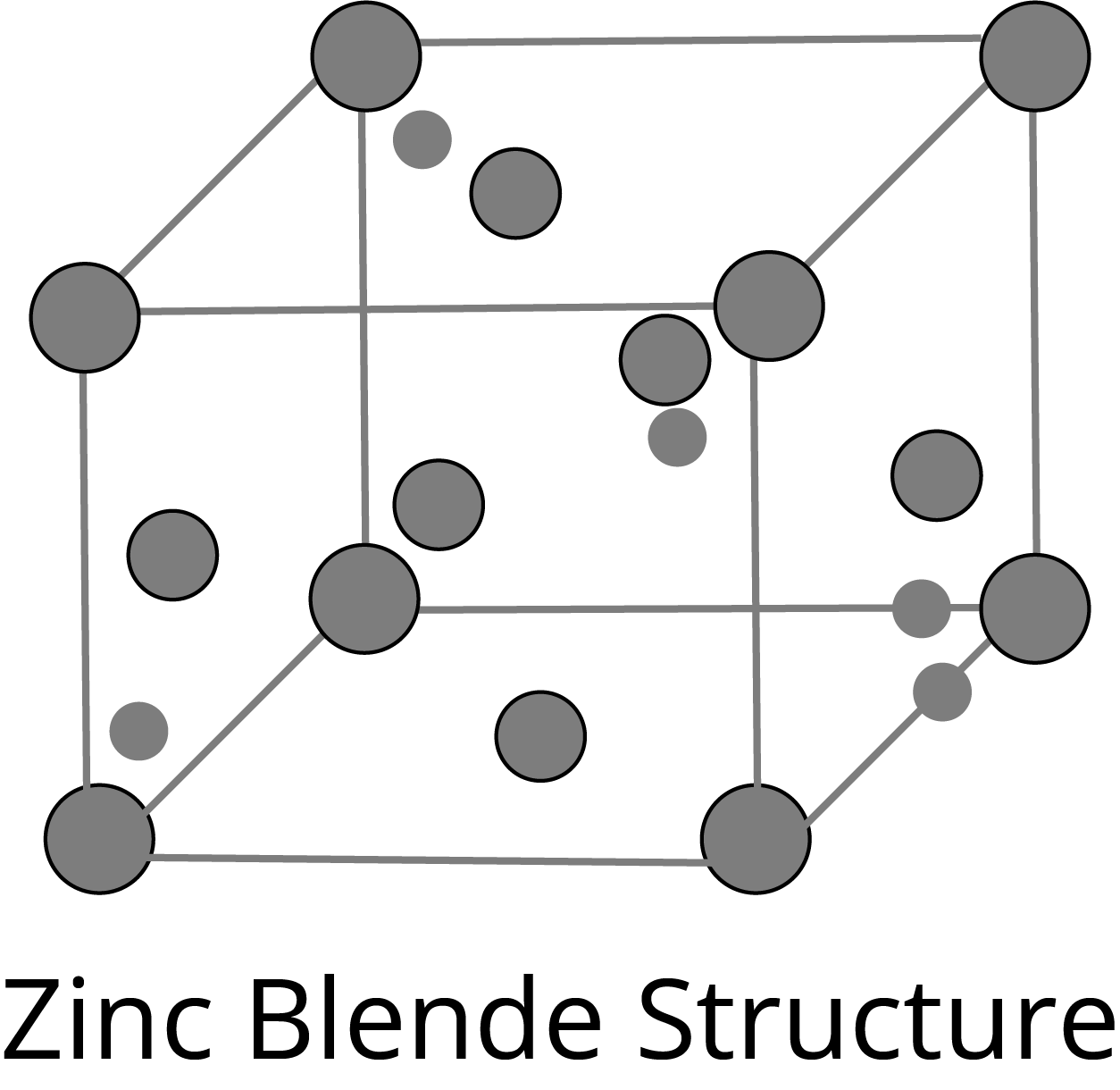S2- ions occupy main position and Zn+2 ions are present in alternate tetrahedral voids in FCC crystal.Effective formula=Zn4S4Co-ordination No. of Zn+2=4Co-ordination No. of S2-=4Distance b/w nearest neigbhours ($r_{Zn^{+2}}+r_{S^{2-}}=\frac{\sqrt{a}}{4}$)

• Fluorite Type Structure:

 Figure Key Points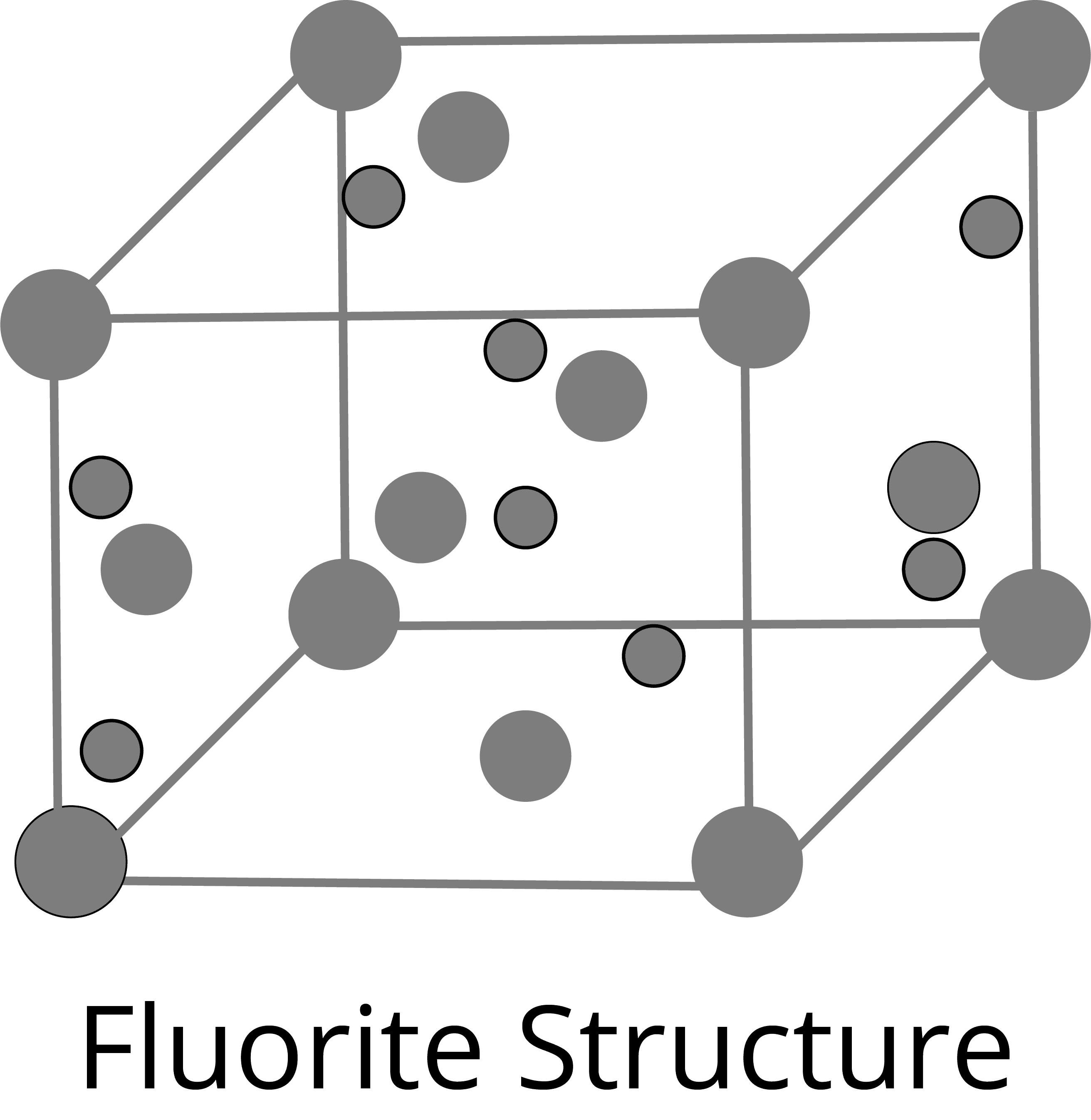Ca2+ ions occupy main position and F- ions are present in tetrahedral voids in FCC crystal.Effective formula=Ca4F8Co-ordination No. of Ca2+=8Co-ordination No. of F-=4Distance b/w nearest neigbhours ($r_{Ca^{+2}}+r_{F^{-}}=\dfrac{a\sqrt{3}}{4}$)

• Anti-fluorite Structure:

 Figure Key Points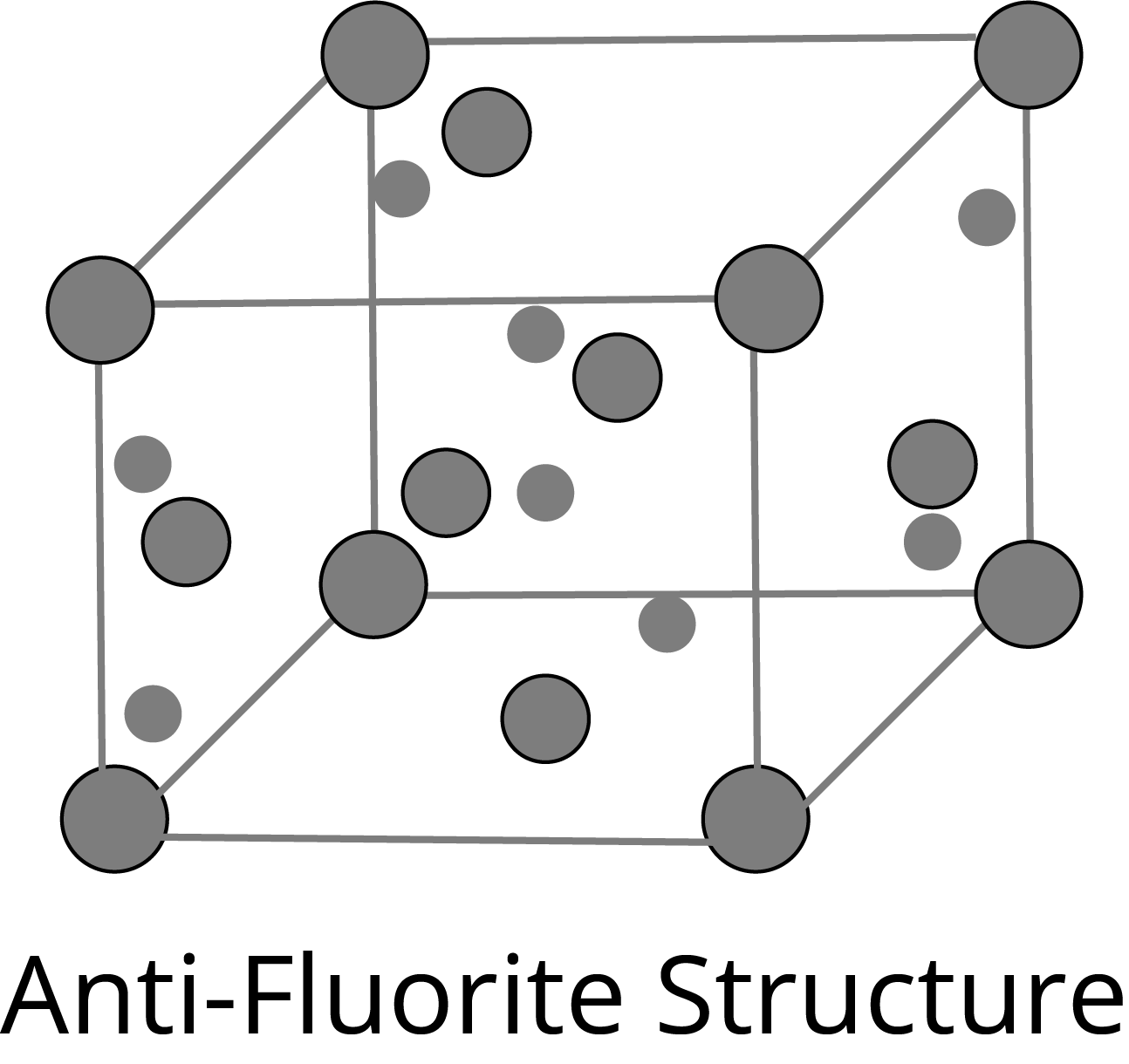Common in alkali oxides like Na2O,Li2O etc.O2- ions occupy FCC Li+ ions Occupy the tetrahedral voids.Co-ordination No. of  Li+ =4Co-ordination No. of O2-=8Distance b/w nearest neigbhours ($r_{Li^{+}}+r_{O^{2-}}=\dfrac{a\sqrt{3}}{4}$)

• CsCl Type Structure:

 Figure Key Points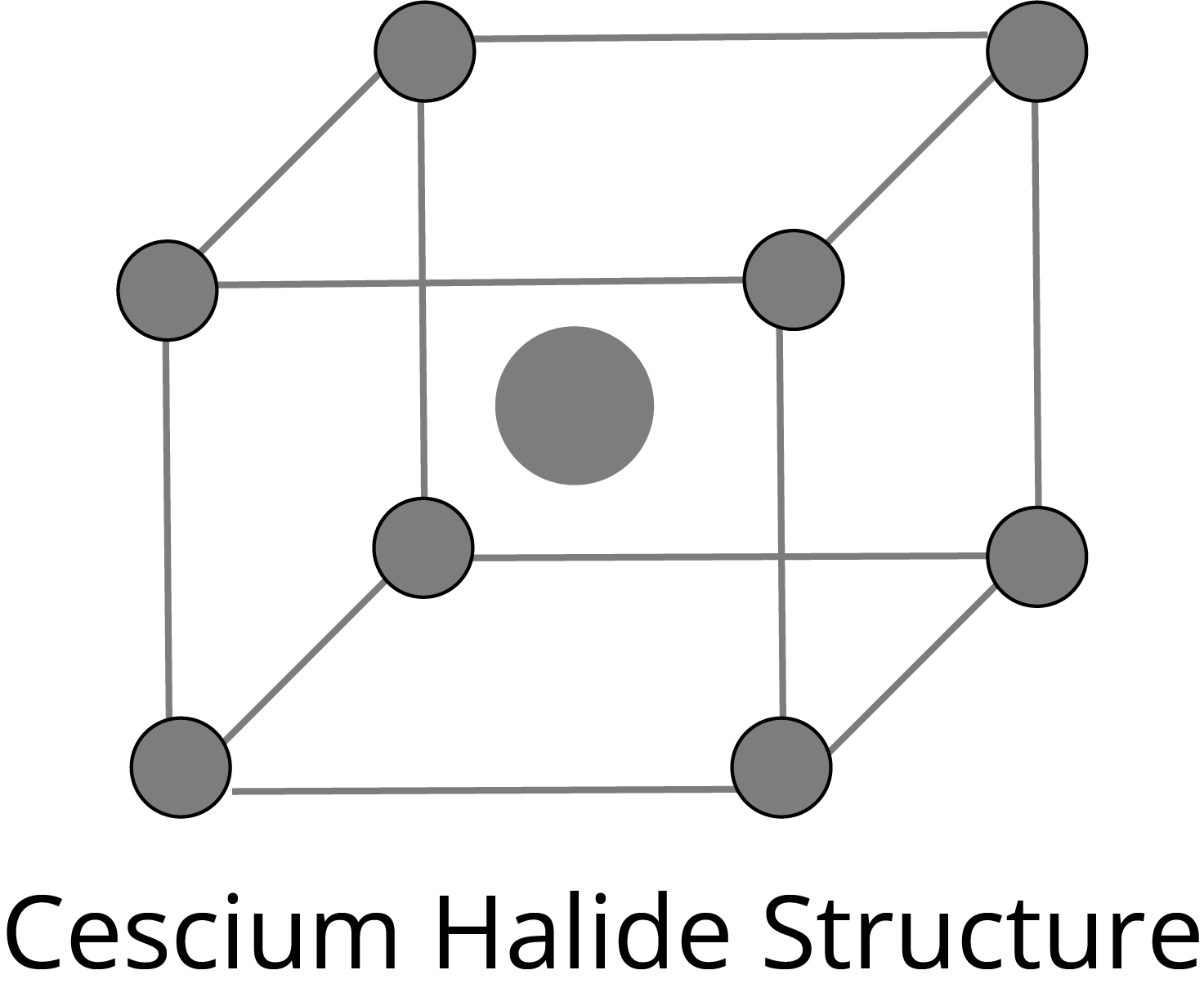Cl- ions simple cubic locations (corners) and Ca+ ions occupy body centre in BCC lattice.Effective formula = CsClCo-ordination No. of Cs+ =8Co-ordination No. of Cl-=8Distance b/w nearest neighbours ($r_{Cs^{+}}+r_{Cl^{-}}=\dfrac{a\sqrt{3}}{2}$)

### Imperfection or Defects in Solids: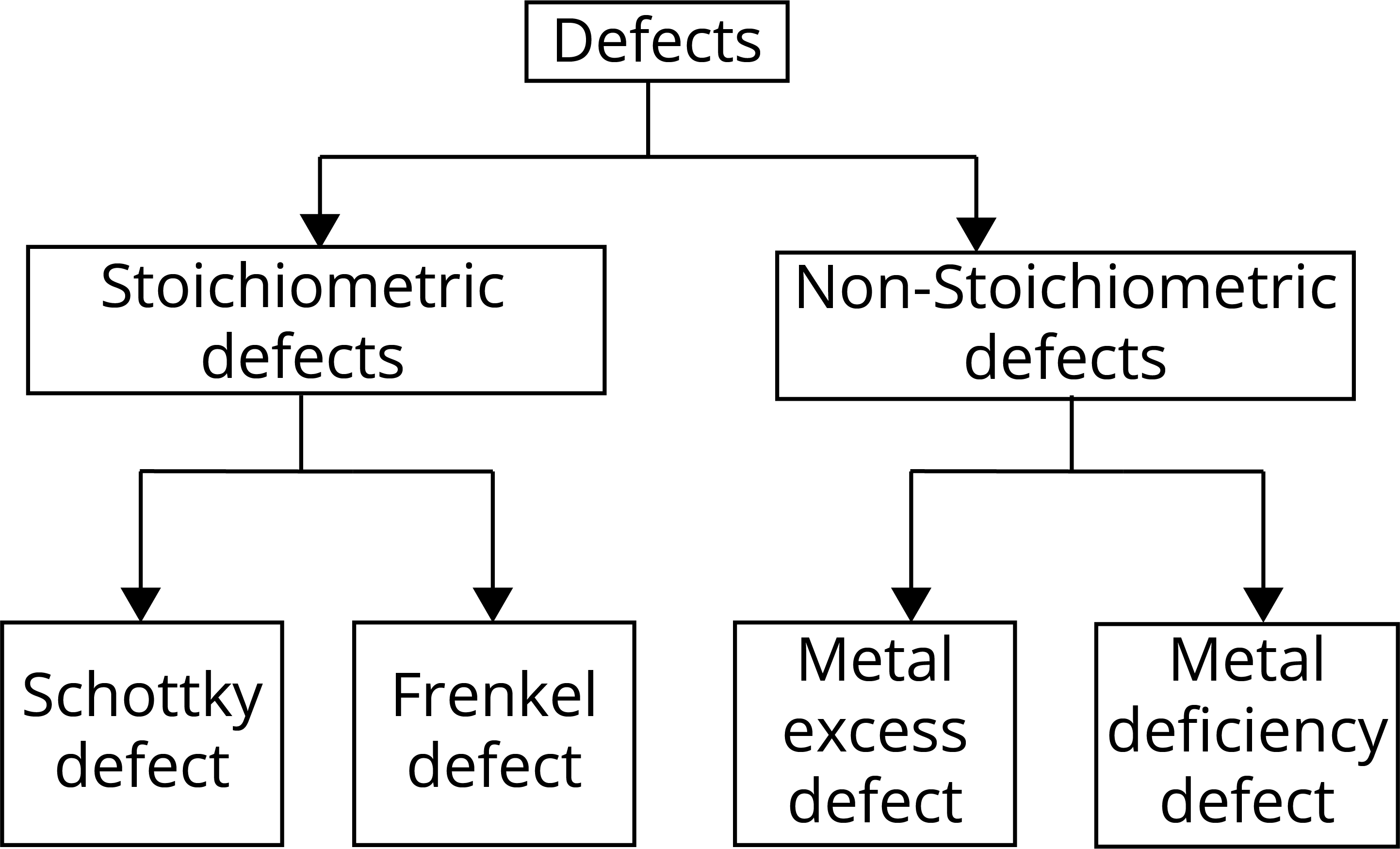1. Stoichiometric Defects: These defects do not change the stoichiometry of the solid. There are two types of stoichiometric defects:

• The Schottky Defect: It is a vacancy defect in which the number of missing cations and anions in the crystal are equal. In this defect, electrical neutrality is maintained but the density of the crystal is decreased. The cations and anions of similar size show this type of defect, for example- KCl, NaCl, AgBr etc.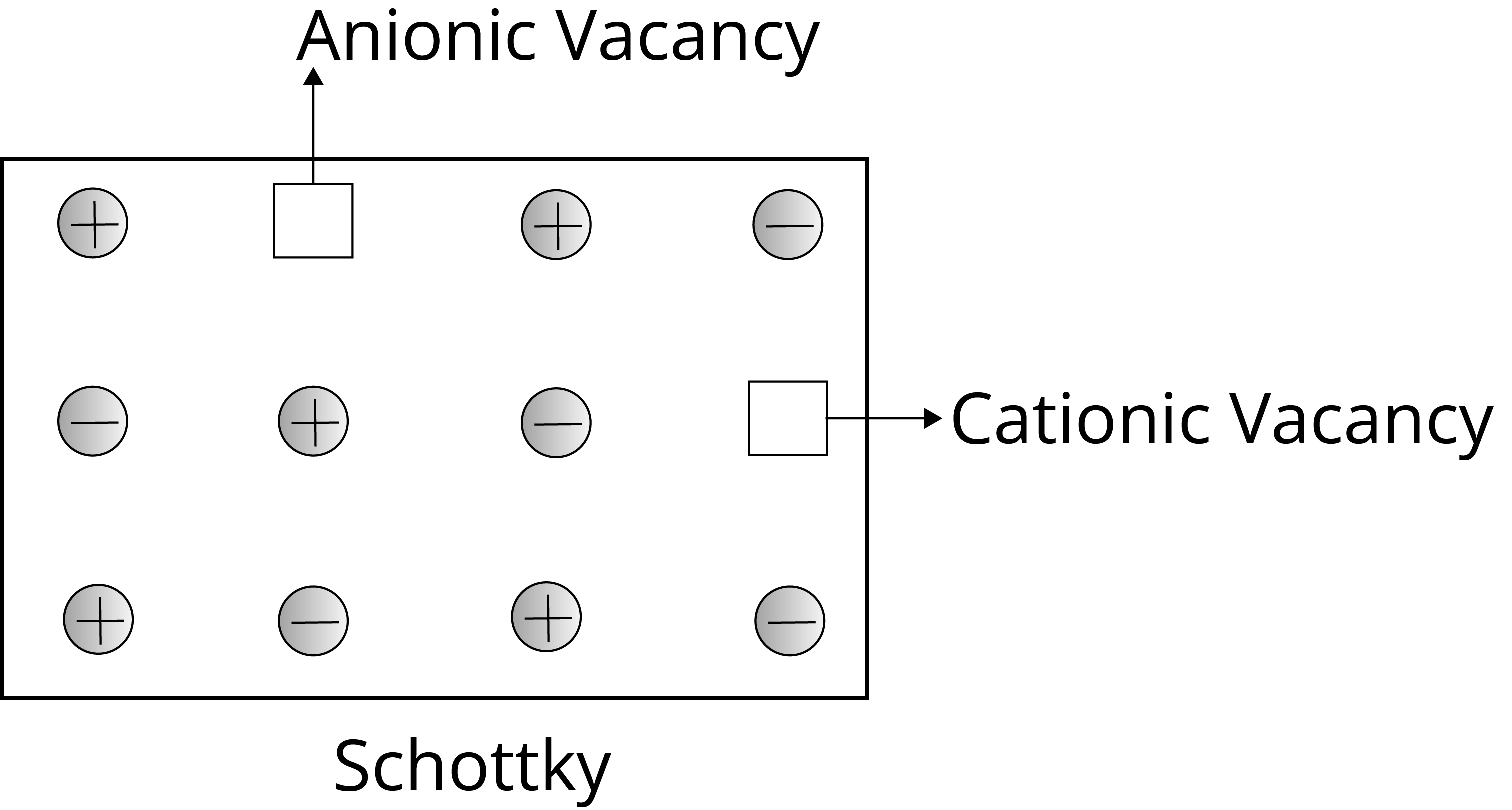• Frenkel Defects: It is the dislocation defect in which the smaller ion is dislocated from its normal position to an interstitial site. The density of the crystal does not change. This type of defect is shown by the ionic solids in which the ions have large differences in size, for example- ZnS, AgCl, AgBr, and AgI.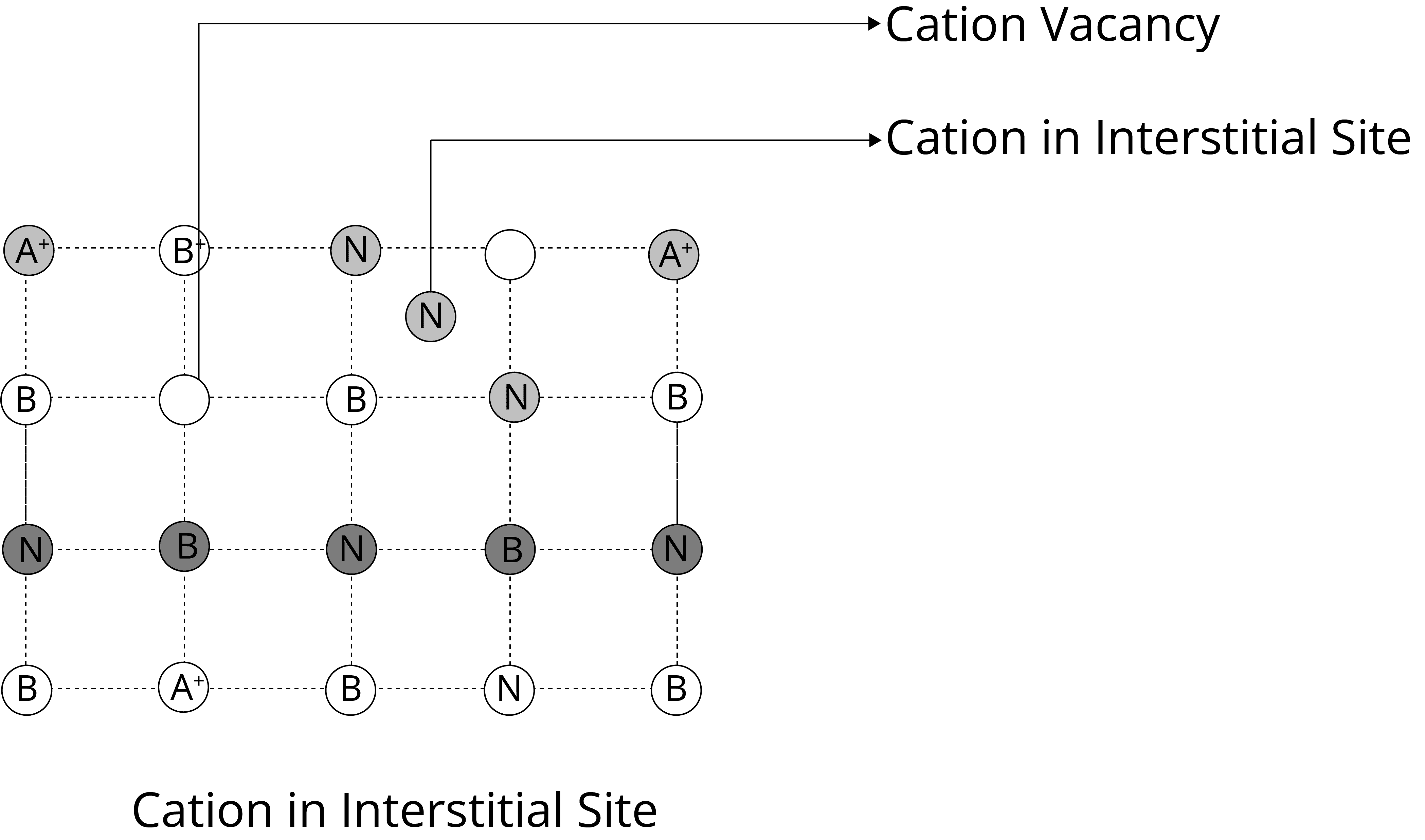2. Non-Stoichiometric Defects: These defects do not change the stoichiometry of the solid. There are two types of non-stoichiometric defects:

• Metal Excess Defect: In this defect, an anion is absent from its lattice position, leaving an electron behind to keep the charge balanced. The site that contains electrons is known as the F centre. They impart colour to the crystal example: NaCl appears yellow, LiCl appears pink and KCl appears lilac (or violet) in excess of their corresponding cationic metal due to metal excess defect.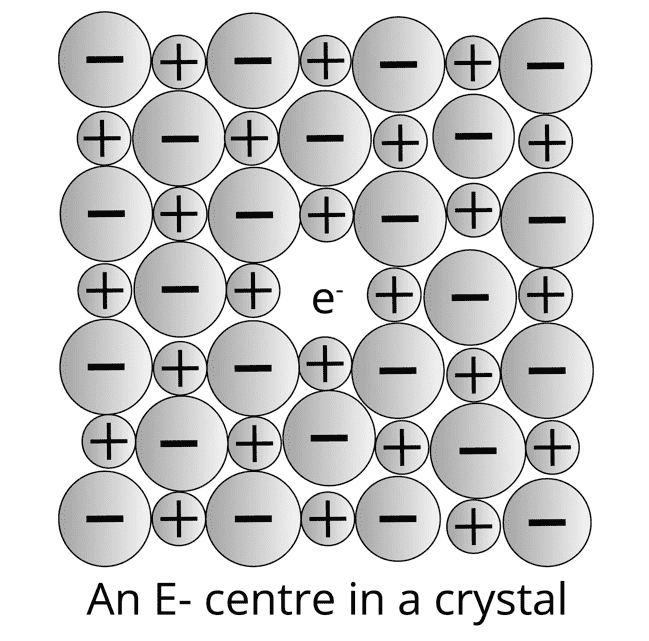• Metal Deficiency Defect: This defect occurs due to variable valency is shown by some metals for example FeO which occur in varying composition Fe0.93O to Fe0.96O. In FeO crystals some of the Fe2+ ion is missing from the crystal and the charge is balanced by Fe3+ ion.

### Solved Examples From the Chapter

Example 1: A metal (atomic mass = 50) has a body-centered cubic crystal structure. The density of the metal is 5.96 g cm-3. Find the volume of the unit cell (NA =  6.023 x 1023 atoms mol-1).  :

1. 1.56  X 10 -20cm3

2. 2.24 X 10-22cm3

3. 2.78 X 10 -23 cm3

4. 5.45 X 10-25cm3

Solution: (c) Given,  M = 50
ρ = 5.96 g cm-
Z = 2 (for BCC)
NA = 6.023 x 1023
V = ?

We know, ρ = $\dfrac{M \times Z}{V \times N_A}$

V   = $\dfrac{M \times Z}{ρ \times N_A}$

= $\dfrac{50 \times 2}{5,96 \times 6.023 \times 10 ^{23}}$

= 2.78  X  10 -23

Key points: Density of cubic crystals is given by formula:

Density,  ρ = $\dfrac{M \times Z}{a^{3}N_A}$

V = a3

Example 2: The atomic radius of nickel is 124 pm. Nickel crystallizes in a face-centered cubic lattice. What is the length of the edge of the unit cell?

1. 430.2 pm

2. 350.7 pm

3. 213.8 pm

4. 620.5 pm

Solution:  (b) Given, r = 124 pm

Edge length, a = ?

$\sqrt{2}$a = 4r

a = 2$\sqrt{2}$r

a  =  2$\sqrt{2}$ X  124 pm  =  350.7 pm

Key Points:

r = $\dfrac{\sqrt{2}a}{4}$

### Solved Questions from the Previous Year Question Papers

Question 1: The parameters of the unit cell of a substance are a = 2.5, b = 3.0, c = 4.0;

α = 90°, β = 120°, γ = 90°. The crystal system of the substance is :

1. Hexagonal

2. Orthorhombic

3. Monoclinic

4. Triclinic

Solution: (c)  According to the question, a ≠ b ≠ c and  α = γ = 90° and β = 120°. The crystal system is monoclinic.

Question 2: A hard substance melts at high temperature and is an insulator in both solid and in molten state. This solid is most likely to be a/an :

1. Covalent solid

2. Molecular solid

3. Ionic solid

4. Metallic solid

Solution: (a) Covalent solids are generally hard substances and melt at very high temperatures. They are insulators in both solid and molten states (except graphite).

Question 3: A diatomic molecule X2 has a body-centred cubic (bcc) structure with a cell edge of 300 pm. The density of the molecule is 6.17 g cm-3. The number of molecules present in 200 g of X2 is

(Avogadro constant (NA)= 6 x 1023 mo1-1

(a) 8 NA

(b) 40 NA

(c) 4 NA

(d) 2 NA

Solution:  (c) Given, a = 300 pm = 3 X 10-8

ρ = 6.17 g cm-3

m = 200 g

Z  =  2(for BCC)

ρ = $\dfrac{M \times Z}{a^{3}N_A}$

6.17  =   $\dfrac{M \times 2}{(3 \times 10^{-8})^{3} \times 10^{23}}$

M  =     50 g / mol

Number of moles = m/M  =  200/50  = 4

Number of molecules = 4 NA

### Practice Questions

Question 1: Which of the following compounds is likely to show both Frenkel and Schottky defects in its crystalline form?

1. CsCl

2. AgBr

3. ZnS

4. KBr

Question 2: The ratio of the number of atoms present in a simple cubic, body-centred cubic and face centred cubic structure are, respectively :

1. 8 : 1 : 6

2. 4 : 2 : 1

3. 1 : 2 : 4

4. 4 : 2 : 3

Answer: (c)  1 : 2 : 4

### Conclusion

In this article, we get to know that solids are more common than liquids and gases in our environment, and we use them more frequently. Solids with a wide range of characteristics are required for various applications. These characteristics are determined by the composition of the constituent particles as well as the binding forces that exist between them. As a result, studying the structure of solids is crucial. The structure and qualities of solids justify their uses for many purposes.

See More## NEET Important Dates

View All Dates
NEET 2022 exam date and revised schedule have been announced by the NTA. NEET 2022 will now be conducted on 17-July-2022, and the exam registration closes on 20-May-2022. You can check the complete schedule on our site. Furthermore, you can check NEET 2022 dates for application, admit card, exam, answer key, result, counselling, etc along with other relevant information.
See More
View All Dates## NEET Information

Application Form
Eligibility Criteria
Reservation Policy
NTA has announced the NEET 2022 application form release date on the official website https://neet.nta.nic.in/. NEET 2022 Application Form is available on the official website for online registration. Besides NEET 2022 application form release date, learn about the application process, steps to fill the form, how to submit, exam date sheet etc online. Check our website for more details.## NEET 2022 Study Material

View NEET Syllabus in Detail
View NEET Syllabus in Detail## NEET 2022 Study Material

View all study material for NEET
All
Physics
Chemistry
Biology
See All## NEET Question Papers## NEET 2022 Book Solutions and PDF Download

View all NEET Important Books
Biology
NCERT Book for Class 12 Biology
Physics
NCERT Book for Class 12 Physics
Chemistry
NCERT Book for Class 12 Chemistry
Physics
H. C. Verma Solutions
See All## NEET Mock Tests

View all mock tests
"NEET 2022 free online mock test series for exam preparation are available on the Vedantu website for free download. Practising these mock test papers of Physics, Chemistry and Biology prepared by expert teachers at Vedantu will help you to boost your confidence to face the NEET 2022 examination without any worries. The NEET test series for Physics, Chemistry and Biology that is based on the latest syllabus of NEET and also the Previous Year Question Papers."
See More## NEET 2022 Cut Off

NEET Cut Off
NTA is responsible for the release of the NEET 2022 cut off score. The qualifying percentile score might remain the same for different categories. According to the latest trends, the expected cut off mark for NEET 2022 is 50% for general category candidates, 45% for physically challenged candidates, and 40% for candidates from reserved categories. For the general category, NEET qualifying marks for 2021 ranged from 720-138 general category, while for OBC/SC/ST categories, they ranged from 137-108 for OBC, 137-108 for SC and 137-108 for ST category.
See More## NEET 2022 Results

The NEET 2022 result is published by NTA on https://neet.nta.nic.in/ in the form of a scorecard on 7-Sep-2022. The scorecard will include the roll number, application number, candidate's personal details, and the percentile, marks, and rank of the candidate. Only those candidates who achieve the NEET cut-off will be considered qualified for the exam.
See More
Rank List
Counselling
Cutoff
NEET 2022 state rank lists will be released by the state counselling committees for admissions to the 85% state quota and to all seats in private medical and dental colleges. NEET 2022 state rank lists are based on the marks obtained in entrance exams. Candidates can check the NEET 2022 state rank list on the official website or on our site.## NEET Top Colleges

View all NEET 2022 Top Colleges
Want to know which Engineering colleges in India accept the NEET 2022 scores for admission to Engineering? Find the list of Engineering colleges accepting NEET scores in India, compiled by Vedantu. There are 1622 Colleges that are accepting NEET. Also find more details on Fees, Ranking, Admission, and Placement.
See More## FAQs on NEET Chapter - The Solid State

FAQ

1. What is solid states class 12?

In the solid-state chapter of class 12, there are properties of solid, types of solids, how they are packed in the crystal and defects in the crystals are discussed.

2. What are the properties of solid states?

The properties of solid states are:

• They have fixed shape, mass and volume.

• The intermolecular force of attraction is strong.

•  The intermolecular distance between the constituent particles of solids is short.

• They are rigid and incompressible.

• They have high density.

3. What is meant by solid-state physics?

Quantum mechanics, crystallography, electromagnetism, and metallurgy are all used in solid-state physics to explore rigid matter or solids. It is the most important subdiscipline in condensed matter physics.## Notice board

News
BlogTrending pages